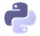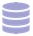# Data Science Courses

Each path is a curated sequence of courses, carefully arranged to take you from zero to job-ready.

### Data Analyst in R

You’ll learn the basics of using R for data analytics, how to work with data sources, data cleaning techniques, how to perform statistical analyses, data visualization techniques, and predictive analysis.

### Data Analyst in Python

You’ll learn the basics of using Python for data analytics, how to work with data sources, data cleaning techniques, how to perform statistical analyses, data visualization techniques, and predictive analysis.### Data Scientist in Python

You’ll learn advanced techniques for using Python for data analytics: working with large datasets, machine learning topics, and the basic computer architecture and parallel processing topics.### Data Engineer

You’ll learn how to work with production databases, how to build data pipelines that can handle larger data sets, and learn key computer science concepts like data structures, algorithms, and recursion.

## Browse Our Data Science Course Catalog

Choose the courses that meet your specific needs. Each of our hands on courses allow you learn through coding so that you pick up concepts quickly.

### Python for Data Science: Fundamentals

Learn the basics of Python programming and data science.

FREE

### Python for Data Science: Intermediate

Learn important tools for your Python data science toolbox.

FREE

### Pandas & NumPy Fundamentals

Learn how to analyze data using the pandas and NumPy libraries.

FREE + BASIC

### Exploratory Data Visualization

Learn how to explore data by creating and interpreting data graphics. This course is taught using matplotlib and pandas.

BASIC

### Storytelling Through Data Visualization

Learn how to communicate insights and tell stories using data visualization.

BASIC

### Data Cleaning and Analysis

Learn how to clean and combine datasets, then practice your skills.

BASIC

### Data Cleaning in Python: Advanced

Learn advanced techniques for cleaning data in Python.

BASIC

### Data Cleaning Project Walkthrough

Learn how to clean and combine datasets, then practice your skills.

BASIC

### Elements of the Command Line

Learn the basics of the Bash to establish a foundation of working the command line as a springboard to using the command line for data science

BASIC

BASIC

### SQL Fundamentals

Learn the basics of working with SQL databases.

FREE

### Intermediate SQL for Data Analysis

Learn to work with multi-table databases.

BASIC

### APIs and Web Scraping in Python

Learn how to acquire data from APIs and the web.

BASIC

### Statistics: Fundamentals

Learn about sampling, variables and distributions.

BASIC

### Statistics Intermediate: Averages & Variability

Learn to summarize distributions, measure variability using variance or standard deviation, and compare values using z-scores.

BASIC

### Probability Fundamentals

Learn the fundamentals of probability theory using Python

BASIC

### Conditional Probability

Learn about conditional probability, Bayes' theorem, and Naive Bayes.

BASIC

BASIC

### Machine Learning Fundamentals

Learn the fundamentals of machine learning using k-nearest neighbors.

### Calculus for Machine Learning

Learn the calculus necessary for intermediate machine learning techniques like linear regression.

### Linear Algebra for Machine Learning

Learn the linear algebra necessary for intermediate machine learning techniques like linear regression.

### Linear Regression for Machine Learning

Learn how to use the linear regression machine learning model.

### Machine Learning in Python: Intermediate

Dive more into Machine learning.

### Decision Trees

Learn how to construct and interpret decision trees.

### Deep Learning: Fundamentals

Learn the basics of deep neural networks. Includes graph representation, activation functions, multiple hidden layers, and image classification.

### Machine Learning Project

Learn what a complete data science project looks like, from data cleaning to machine learning.

### Kaggle Fundamentals

Learn how to get started with and participate in Kaggle competitions with Kaggle's 'Titanic' competition.

Learn how to write high-quality functions.

BASIC

### Git & Version Control

Learn the basics of Python programming and data science.

BASIC

### Spark & Map-Reduce

Learn how to use Apache Spark and the map-reduce technique to clean and analyze large datasets.

### Python Fundamentals

Learn the basics of Python programming for data engineering.

FREE

### Python Intermediate

Learn important tools for your Python data science toolbox.

FREE

### Programming Concepts with Python

Enhance your understanding of how Python works.

### SQL Intermediate: Table Relations & Joins

Learn to work with multi-table databases.

### Postgres for Data Engineers

Learn about the SQL database Postgres.

### Optimizing Postgres Databases

Learn how to optimize your Postgres databases.

### NumPy for Data Engineers

Learn to optimize your data processing using the NumPy library.

### Processing Large Datasets in Pandas

Learn how to work with datasets by optimizing your pandas workflow, processing data in batches, and augmenting pandas with SQLite.

### Parallel Processing

Learn parallel processing and MapReduce.

### Data Structures Fundamentals

Learn the fundamentals of data structures — Linked Lists, Queues, Stacks and Dictionaries, etc.

### Recursion and Trees

Learn about recursion and how it applies to tree data structures, and how tree data structures are used to speed up processing of data analysis tasks.

### Building a Data Pipeline

Learn how to build a Python data pipeline from scratch.

### Introduction to Data Analysis in R

Learn the basics of R, a popular programming language for data analysis.

FREE

### Data Structures in R

Learn about vectors, matrices, lists, dataframes, and more in R.

FREE

### Control Flow, Iteration, and Functions in R

Enhance your R programming skills with if statements, for loops, and much more.

FREE

### Specialized Data Processing in R: Strings and Dates

Learn to work with specialized data types like text data, times, and dates in R.

FREE

### Data Visualization in R

Learn to use the ggplot2 package for exploratory data visualization in R.

BASIC

### Data Cleaning in R

Learn to perform common data cleaning tasks.

BASIC

### Data Cleaning in R: Advanced

Learn advanced techniques for cleaning data in R.

BASIC

### Intermediate SQL in R

Learn to work with multi-table databases.

BASIC

### APIs in R

Learn to work with APIs in R.

BASIC

### Web Scraping in R

Learn to scrape data from webpages using R and rvest.

BASIC

### Statistics Fundamentals in R

Learn about sampling, variables and distributions.

BASIC

### Statistics Intermediate in R: Averages and Variability

Learn some intermediate statistic techniques such as calculating z-scores.

BASIC

### Probability Fundamentals in R

Learn the fundamentals of probability theory using R.

BASIC

### Conditional Probability in R

Learn about Bayes's Theorem and use it to build a spam filter.

BASIC

### Hypothesis Testing in R

Learn the fundamentals of hypothesis testing using R.

BASIC

### Linear Regression Modeling In R

Learn linear regression modeling in R.

BASIC

### Introduction to Machine Learning in R

Learn the fundamentals of machine learning using R and the caret library.

BASIC